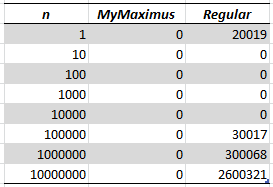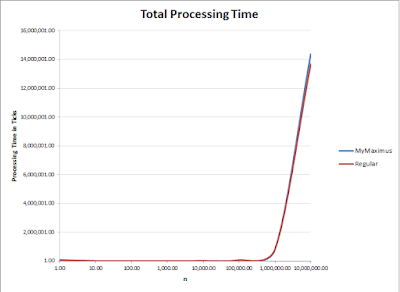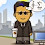## Sunday 15 November 2015

### Reading the Maximum of an Array

There's an interesting question on Quora doing the rounds at the moment. It's entitled:

"What's the fastest algorithm to find the largest number in an unsorted array?"

To repeatedly find the largest value of any array supplied to a function is O(N). You have to look at each element once, since you're checking if it is a new maximum. However can we do better?

I postulated this left of field idea in response to a comment from the original poster, which stated their lecturer asked them "what is it that an array automatically has which may be used to improve on this?"

"How about setting the array 'Base' address (pointer to the address of the first item)  to the largest number? After all, all you want is the one dereferenced pointer value. Not to sort or search the array. So you're then effectively always reading the value at address array. Therefore O(1) each time"

In principle, this is the same as other answers appearing which propose storing the index of the maximum location as you build the array.

### The Building

Building an array somewhere is at least an O(1) operation. This lower bound is defined using the Omega asymptotic notation. So

$\Omega(1)$

Here is where it gets interesting. Normally, to find the maximum value of an array by iterating through each element, we'd get a $O(n)$ algorithm as we iterate through each item and compare it to our maximum. i.e. the algorithm (in C#):

public static int Maxi(ArrayList array)
{
var maxValue = 0;
for(var i = 0; i < array.Count; i++)
{
if ((int)array[i] > maxValue)
maxValue = (int)array[i];
}

return maxValue;
}

Is an $O(n)$ algorithm.

### Efficiency Step

My proposal was essentially to create the efficiency, by the combination of the two operations into one. So you would build the array but keep hold of the maximum value as you build it. You can encapsulate it in a class, we'll call it MyMaximus, just for fun and it is essentially:

public class MyMaximusArray
{
public int Maximum { get; private set; }

private MyMaximusArray()
{
}

public static MyMaximusArray CreateFrom(int arrayLength)
{
var result = new MyMaximusArray();
var random = new Random();
var array = new ArrayList(arrayLength);

for (var i = 0; i < arrayLength; i++)
{
var value = random.Next(int.MaxValue);

if (value > result.Maximum)
result.Maximum = value;
}

return result;
}
}

Now, just reading the Maximum property gives you the maximum value. You can alternatively substitute the value of Maximum to be the index in the static function and you have what I was suggesting.

The expectation is you'd substitute a reduction in array build time on building for the read time when locating the maximum item in the array. This is particularly suitable where the data doesn't change.

### How Much Quicker?

So, the proof of the pudding is in the eating. Hence, I wrapped the two of these this up in a little console app with a tick-timer which read the ticks at the start and end and output the result, including for the sub-phases of building the array and reads of the maximum. For those people unfamiliar with ticks, they are a measure of 1/10,000 of a millisecond (1/10,000,000) which is sufficient for most applications.

The tests were each run three times, over a logarithmic scale from 1 to 10,000,000 and an average taken across the three.

The algorithms were then modified to behave as if they would be written to once and the maximum read many times.

The results were pretty conclusive:

#### Build Time

The expectation would be the 'search optimised' algorithm (MyMaximus) would perform worse than the regular algorithm when first building the array and sure enough it did, though surprisingly, not as much I thought. Both algorithms on this stage of the process would be O(n), with only a difference in coefficient. The 'doubling' due to the introduction of the if comparison didn't quite occur, though I speculate this may be due to JIT optimisation on the .NET platform.

#### Maximum Value Search

Here is where the MyMaximus version was expected to make the gains according to the maths. This also behaved as expected:

The blue line is following the axis because it genuinely was zero. Here are the actual data points:Maximum item search times (ticks)

The reason it is zero is that I am running this on a 4 GHz system, with a 20x clock multiplier and 1866MHz ram. All in all, this means it can carry out a CPU cycle including memory access (the slowest part of this process) therefore one read instruction occurs every 0.000000000526 of a second, which if a tick is 0.0000001 of a second, will never register. Hence, this result.

#### Total Time

The combination of build and run fulfils the full scenario. Here, we expected the MyMaximus read to achieve similar asymptotic performance on the single run, but perform substantially better on the continual searches, tending towards $\Omega(n)$ the more searches that happened.single run comparison

### Total Search Performance by Size of Array

So overall, the performance of MyMaximus versus a regular search resulted in a small and I'd argue insignificant (chi-squared another day) win in the single search case. What happens in the case of the array being queried for it's maximum multiple times? The expectation is that the average will start off about the same when building the array, but the performance of the queries will be much faster with MyMaximus.

To test this, I created the same setup, but this time asking for the maximum 1000 times per array size. The end results seemed to live up to that expectation:

So what exactly happens?

It's pretty straightforward. The lower bound of the MyMaximus algorithm is $\Omega(n)$ whilst the lower bound of the regular algorithm is  $O(n)$ and that's the best you can do. So MyMaximus tends to the lower bound over time, whilst the regular algorithm does not.

### Conclusion

The context of a particular algorithm is as important to the asymptotic complexity as the individual optimisation itself. This is well a known idea in Electronic engineering, as logic circuitry, such as NAND gate representaitons of other circuitry, are often evaluated to remove unnecessary gates and save money. Here you're doing it to save time, which in this era of cloud computing, also happens to save you money and indeed, losses if customers are not put off.

In any case, the question on Quora had an academic slant. Just be aware there's more there than meets the eye :)

1.Great analysis on something people should do as a matter of course, if the array is of their own creation. I would file this under "no-brainers" - although I'm sorry to say it will completely fall apart if you remove an item from the array or modify an item so that it is LESS THAN the already-established maximum, since you'll need to reevaluate the whole array to find out the maximum. This also falls apart if you're receiving the array from the other side of a method call (be that over a service boundary, process boundary or even just across classes each having their own strict Single Responsibility) - in those cases you might state in the Operation Contract that you want the maximum, figure it out once when the array is received or use an on-demand caching system.

2.Spot on, and I did cover this in the answers to the Quora question, which has got rather large now.

https://www.quora.com/What-is-the-fastest-algorithm-to-find-the-largest-number-in-an-unsorted-array

I should probably copy and paste here. The only context where it is guaranteed to affect the O(1) read it is the deletion or change of the maximum itself.

If you delete at a point that isn't the maximum, then the value hasn't changed, so no need to recalculate.

If you insert a new value, then you compare against the maximum on insertion (one if statement, so still O(1)) and reset the maximum to it if needed.

However, if you delete/modify the maximum, as you say Hugh, you have to recalibrate it. Equally, if you pass a new array altogether, you have to find the maximum there too. These are O(N) operations, but if there are 10,000 reads per 1 of these, then the analysis in the article still applies equally.

The key to this all is context and without knowing if the context is entirely delete biased, you can't really make the decision to use this or not. I envisaged the MyMaximus class being immutable, given what they wanted it to do. A real world scenario would implement the .NET IList (or ICollection if an external index accessible item isn't needed) and override the CRUD methods to deal with handling the maximum value.